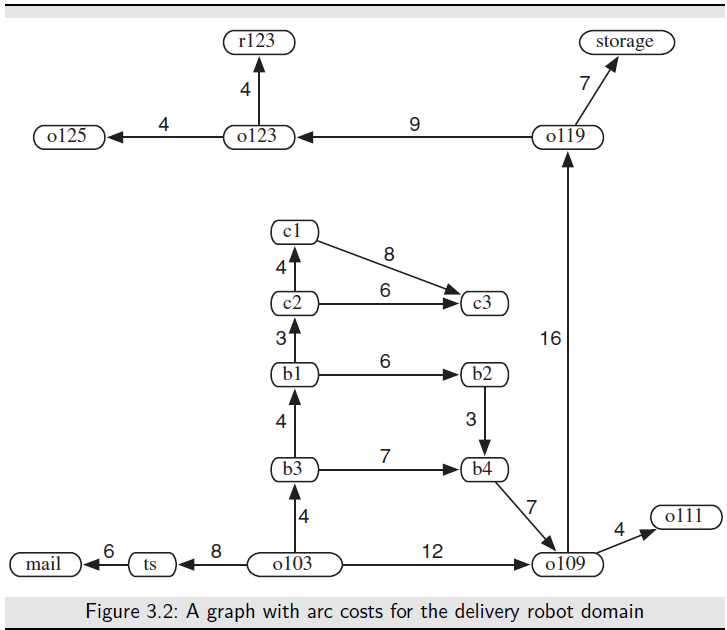Graph Searching: In this section, we abstract the general mechanism of searching and present it in terms of searching for paths in directed graphs. To solve a problem, first define the underlying search space and then apply a search algorithm to that search space. Many problem-solving tasks can be transformed into the problem of finding a path in a graph. Searching in graphs provides an appropriate level of abstraction within which to study simple problem solving independent of a
particular domain.

A (directed) graph consists of a set of nodes and a set of directed arcs between nodes. The idea is to find a path along these arcs from a start node to a
goal node. The abstraction is necessary because there may be more than one way to represent a problem as a graph. Whereas the examples in this chapter are in terms of state-space searching, where nodes represent states and arcs represent actions, future chapters consider different ways to represent problems as graphs to search.

Formalizing Graph Searching: A directed graph consists of

• a set N of nodes and
• a set A of ordered pairs of nodes called arcs.

In this definition, a node can be anything. All this definition does is constrain arcs to be ordered pairs of nodes. There can be infinitely many nodes and arcs. We do not assume that the graph is represented explicitly; we require only a procedure that can generate nodes and arcs as needed. The arc n1, n2 is an outgoing arc from n1 and an incoming arc to n2. A node n2 is a neighbor of n1 if there is an arc from n1 to n2; that is, if n1, n2 ∈ A. Note that being a neighbor does not imply symmetry; just because n2 is a neighbor of n1 does not mean that n1 is necessarily a neighbor of n2. Arcs may be labeled, for example, with the action that will take the agent from one state to another.

A path from node s to node g is a sequence of nodes n0, n1, . . . , nk such that s = n0, g = nk, and ni−1, ni ∈ A; that is, there is an arc from ni−1 to ni for each i. Sometimes it is useful to view a path as the sequence of arcs, no, n1 , n1, n2 , . . . , nk−1, nk, or a sequence of labels of these arcs. A cycle is a nonempty path such that the end node is the same as the start node – that is, a cycle is a path n0, n1, . . . , nk such that n0 = nk and k = 0. A directed graph without any cycles is called a directed acyclic graph (DAG). This should probably be an acyclic directed graph, because it is a directed graph that happens to be acyclic, not an acyclic graph that happens to be directed, but DAG sounds better than ADG!

A tree is a DAG where there is one node with no incoming arcs and every other node has exactly one incoming arc. The node with no incoming arcs is called the root of the tree and nodes with no outgoing arcs are called leaves. To encode problems as graphs, one set of nodes is referred to as the start nodes and another set is called the goal nodes. A solution is a path from a start node to a goal node.Sometimes there is a cost – a positive number – associated with arcs. We write the cost of arc ni, nj as cost( ni, nj ). The costs of arcs induces a cost of paths. Given a path p = n0, n1, . . . , nk, the cost of path p is the sum of the costs of the arcs in the path:
cost(p) = cost(n0, n1) · · · cost(nk−1, nk)
An optimal solution is one of the least-cost solutions; that is, it is a path p from a start node to a goal node such that there is no path p from a start node to a goal node where cost(p) < cost(p).

In many problems the search graph is not given explicitly; it is dynamically constructed as needed. All that is required for the search algorithms that follow is a way to generate the neighbors of a node and to determine if a node is a goal node.
The forward branching factor of a node is the number of arcs leaving the node. The backward branching factor of a node is the number of arcs entering the node. These factors provide measures of the complexity of graphs. When we discuss the time and space complexity of the search algorithms, we assume that the branching factors are bounded from above by a constant.

The branching factor is important because it is a key component in the size of the graph. If the forward branching factor for each node is b, and the graph is a tree, there are bn nodes that are n arcs away from any node.